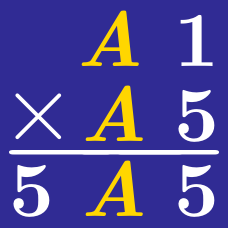Logic

# Cryptogram - Problem Solving

$\large{\begin{array}{ccccccc} && & & & A &B&C\\ && & & & & A&B\\ +&& & & & & &A\\ \hline && & & & 8 & 7&6 \end{array}}$

Find the value of the 3-digit integer $\overline{ABC}$.

$\large{\begin{array}{ccccccc} && & & & &&X\\ && & & & & Y&X\\ +&& & & & Z &Y &X\\ \hline && & & & 4 & 3&2 \end{array}}$

Let $X,Y$ and $Z$ be distinct digits that satisfy the cryptogram above.

Find the value of the 3-digit integer $\overline{ZYX}$.

$\large{\begin{array}{ccccccc} && & & & & 3& L \\ \times && & & & &M &M\\ \hline & & & & 2&N & 6&4 \end{array}}$

Find the value of $L$.

Hint: Using the divisibility rule for 11.

$\large{\begin{array}{ccccccc} && & &T & U& V&W\\ \times&& & & & &9&9\\ \hline & & T & U & U& 5& 5&1 \end{array}}$

Find the value of $T.$

Hint: 99 = 100 - 1.

$\begin{array}{ccccc} & & & & &X\\ & & & &X &Y\\ + & & & Z & Y &Z \\ \hline & & & Y & X &Z \end{array}$

Solve the cryptogram above, where $X,Y,Z \ne 0$, and find the value of the 3-digit integer $\overline{YXZ}$.

×